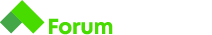# Converter problem python

Здравейте, имам проблем със задачата за конвертиране на MPG to Km/l. Ако някой може да ме ориентира къде бъркам
Задача:

# Converter

You want to buy this really cool car from the UK, but you are worried about the fuel consumption. The values you see are MPG (miles per gallon). You have no idea what 20 MPG means so, being a programmer, decide to write a converter that helps you calculate the consumption.

Doing some research, you learn that `1 gallon = 4.54 litres` and `1 mile = 1.6 km` .

After the calculation, round the result down to the neareast whole number.

## Input

• On the first line you will receive a number m - miles per galon

## Output

• On the only line of output, print `{result} litres per 100 km`

## Constraints

• 1 <= m <= 100

## Input

``````20
``````

## Output

``````14 litres per 100 km
``````

## Input

``````44
``````

Мое решение:

a_20miles_per_galon = int(input())

one_liter = 4.54
one_kilometer = 1.6

kilometers = a_20miles_per_galon * one_kilometer

litres = one_liter / kilometers

litres_sum = litres * 100

print(round(litres_sum), “litres per 100 km”)

1 Like

Привет!
Не трябва ли да се закръгли надолу /с math.floor()/?

1 Like

Да , благодаря получи се :))

1 Like